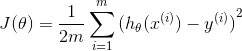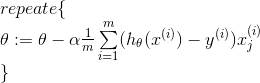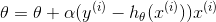# 【机器学习】—各类梯度下降算法 简要介绍

### 目录

batch、mini-batch、SGD、online的区别在于训练数据的选择上：``for epoch in range(epoches):y_pred = np.dot(weight, x_train.T)deviation = y_pred - y_train.reshape(y_pred.shape)gradient = 1/len(x_train) * np.dot(deviation, x_train)weight = weight - learning_rate*gradient``

# 二、随机梯度下降(SGD)``for epoch in range(epoches):for i in range(len(x_train):index = random.randint(0,len(x_train))y_pred = np.dot(weight, x_train[index].T)deviation = y_pred - y_train[index].reshape(y_pred.shape)gradient = np.dot(deviation, x_train[index])weight = weight - learning_rate*gradient``

# 三、小批量随机梯度下降（Mini Batch Stochastic Gradient Descent）

``def batch_generator(x, y, batch_size): //batch生成器nsamples = len(x)batch_num = int(nsamples / batch_size)indexes = np.random.permutation(nsamples)for i in range(batch_num):yield (x[indexes[i*batch_size:(i+1)*batch_size]], y[indexes[i*batch_size:(i+1)*batch_size]])for epoch in range(epoches):for x_batch, y_batch in batch_generator(X_train, y_train, batch_size):y_hat = np.dot(weight, x_batch.T)deviation = y_hat - y_batch.reshape(y_hat.shape)gradient = 1/len(x_batch) * np.dot(deviation, x_batch)weight = weight - learning_rate*gradient``

# 四、Online GD

Online GD于mini-batch GD/SGD的区别在于，所有训练数据只用一次，然后丢弃。这样做的好处是可以最终模型的变化趋势。比如搜索广告的点击率(CTR)预估模型，网民的点击行为会随着时间改变。用batch算法（每天更新一次）一方面耗时较长（需要对所有历史数据重新训练）；另一方面，无法及时反馈用户的点击行为迁移。而Online Leaning的算法可以实时的最终网民的点击行为迁移。

1.https://blog.csdn.net/qq_40765537/article/details/105792978
2.https://www.cnblogs.com/richqian/p/4549590.html
—————————————————————————————————————————————————